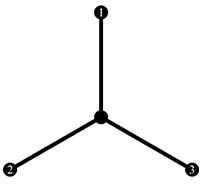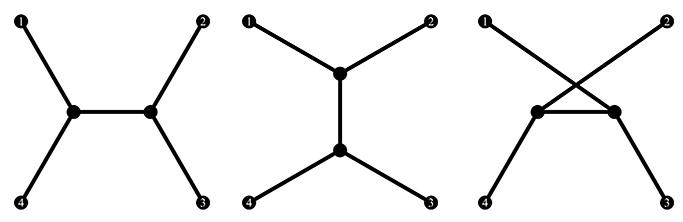favorite Saytın davamlılığını təmin etmək üçün sizin kəməyinizə ehtiyacımız vardır, ətrafli məlumat üçün bannerə klikləyin
Məsələlər

# Full Steiner Topologies

A full Steiner topology for a given point set P = {p1,p2,,pn} is an undirected tree T = (V,E) where V = {v1,v2,,vn,vn+1,,v2n-2} is the set of vertices, and E is the set of edges. The n distinctly labeled leaves of T, v1, v2, , vn, correspond to p1, p2, , pn, respectively; the remaining n - 2 vertices, vn+1, vn+2, , v2n-2, called the Steiner vertices, are mutually indistinguishable and each have a degree of three. Figure 1 shows the only full Steiner topology for P = {p1, p2, p3}. Figure 2 shows all three different full Steiner topologies for P = {p1,p2,p3,p4}.Figure 1: Full Steiner topology for P = {p1, p2, p3}Figure 2: Full Steiner topologies for P = {p1,p2,p3,p4}

Given n, the cardinality of P, compute the number of distinct full Steiner topologies.

Input

The input contains multiple test cases. Each test case consists of a single integer n (3n107) on a separate line. The input ends where EOF is met.

Output

For each test case, print the answer on a separate line. You shall print the answer rounded to four significant digits. Let m · 10e be the scientific form of the rounded answer, you shall print "mEe", giving all four significant digits of m and stripping any leading zeroes before e.

Zaman məhdudiyyəti 1 saniyə
Giriş verilənləri #1
```3
30
300
3000
30000
300000
3000000
```
Çıxış verilənləri #1
```1.000E0
8.687E36
5.677E697
1.462E10024
1.983E130306
4.215E1603145
7.937E19031556
```